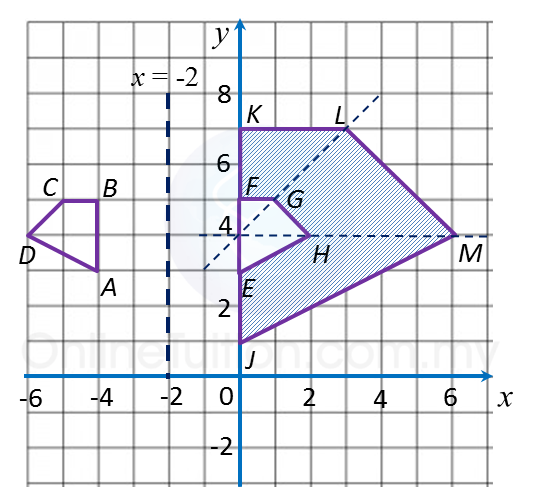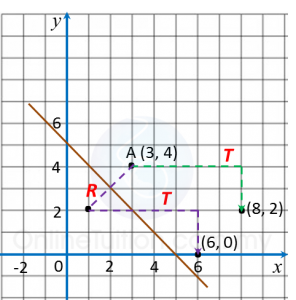# 5.5.1 Combined Transformation, SPM Paper (Long Questions)

Question 1:
(a) Transformation is a translation $\left(\begin{array}{l}-4\\ \text{}2\end{array}\right)$ and transformation P is an anticlockwise rotation of 90oabout the centre (1, 0).

State the coordinates of the image of point (5, 1) under each of the following transformation:
(i) Translation T,
(ii) Rotation P,
(iii) Combined transformation T2.

(b) Diagram below shows three quadrilaterals, ABCD, EFGH and JKLM, drawn on a Cartesian plane.(i) JKLM is the image of ABCD under the combined transformation VW.
Describe in full the transformation:
(a) W   (b) V

(ii) It is given that quadrilateral ABCD represents a region of area 18 m2.
Calculate the area, in m2, of the region represented by the shaded region.

Solution:
(a)(b)(i)(a)
W: A reflection in the line x = –2

(i)(b)
V: An enlargement of scale factor 3 with centre (0, 4).

(b)(ii)
Area of EFGH = area of ABCD = 18 m2
Area of JKLM = (Scale factor)2 × Area of object
= 32 × area of EFGH
= 32 × 18
= 162 m2
Therefore,
= Area of JKLM – area of EFGH
= 162 – 18
= 144 m2

Question 2:
(a) Diagram below shows point A and straight line y + x = 5 drawn on a Cartesian plane.Transformation T is a translation
Transformation R is a reflection at the line y + x = 5.

State the coordinates of the image of point A under each of the following transformations:
(i) Transformation T,
(ii) Combined transformation TR.

(b) Diagram below shows pentagons JKLMN, PQRST and PUVWX, drawn on a Cartesian plane.(i) PUVWX is the image of JKLMN under the combined transformation CB.
Describe in full the transformation:
(a) B    (b) C

(ii) It is given that pentagon JKLMN represents a region of area 80 m2 .
Calculate the area, in m2 , of the region represented by the shaded region.

Solution:
(a)(i)
(3, 4) → T → (8, 2)
(ii)
(3, 4) → R → (1, 2) → T → (6, 0)

(b)(b)(i)(a)
B:
A clockwise rotation of  90o about the centre (0, 2).

(b)(i)(b)

(b)(ii)
Area of PQRST = Area of JKLMN
= 80 m2